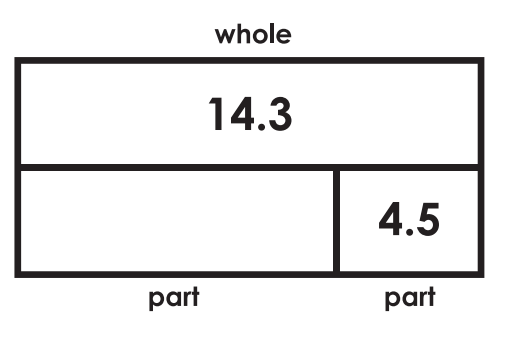Addition of Decimals
Subtraction of Decimals
Multiplication of Decimals
Division of Decimals
Potpourri

3.2 + 5.9 =

9.1

2.9 - 0.4 =

2.5

3.2 x 8 =

25.6

7.2 / 6 =

1.2

### 1009.8

1.54 + 12.4 =

13.94

79.09 - 54.18 =

24.91

13.04 x 4 =

52.16

2.4 / 8

0.3

### 200

A baker uses 5.5 pounds of flour daily. How many pounds of flour will he use in two weeks?

38.5 or 27.5 pounds

134.09 + 98.5 +

232.59

4.05-0.024 =

4.026

7.9 x 28 =

221.2

4.628 / 52 =

0.089

### 300

For a field trip, the school bought 47 sandwiches for $4.60 each and 39 bags of chips for$1.25 each.
How much did the school spend in all?

\$33.75

10.09 + 984 =

994.09

34 - 0.04 =

33.96

9.048 x 62 =

560.976

228.15 / 45 =

5.07

### 500

Twins Bakery has 12 bags of flour in their bakery. Each bag weighs 4.53 pounds. They will use all of the flour to make 18 batches of cinnamon rolls. Each batch of rolls uses an equal amount of flour. How many pounds of flour will they use for each batch?

3.02 pounds of flour for each batch.